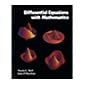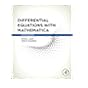Normal view

# Differential equations with Mathematica [electronic resource] / Martha L. Abell, James P. Braselton.

Material type:TextPublisher: Amsterdam ; Boston : Elsevier Academic Press, �2004Edition: 3rd edDescription: 1 online resource (xvi, 876 pages) : illustrationsContent type: text Media type: computer Carrier type: online resourceISBN: 9780080521794; 0080521797Genre/Form: Electronic books.Additional physical formats: Print version:: Differential equations with Mathematica.DDC classification: 515/.35/02855369 LOC classification: QA371.5.D37 | A24 2004ebOnline resources: Click here to access online
Contents:
Front Cover; Differential Equations with Mathematica; Copyright Page; Contents; Preface; Chapter 1. Introduction to Differential Equations; Chapter 2. First-Order Ordinary Differential Equations; Chapter 3. Applications of First-Order Ordinary Differential Equations; Chapter 4. Higher-Order Differential Equations; Chapter 5. Applications of Higher-Order Differential Equations; Chapter 6. Systems of Ordinary Differential Equations; Chapter 7. Applications of Systems of Ordinary Differential Equations; Chapter 8. Laplace Transform Methods; Chapter 9. Eigenvalue Problems and Fourier Series.
Summary: The Third Edition of the Differential Equations with Mathematica integrates new applications from a variety of fields, especially biology, physics, and engineering. The new handbook is also completely compatible with recent versions of Mathematica and is a perfect introduction for Mathematica beginners. The book/CD-ROM package contains built-in commands that lets the user solve problems directly using graphical solutions.
List(s) this item appears in: Mathematica
Tags from this library: No tags from this library for this title.Average rating: 0.0 (0 votes)
Item type Current location Collection Call number Status Date due Barcode Item holdseBook e-Library

Electronic Book@IST

ebooks Available
Total holds: 0

Includes bibliographical references (pages 867-868) and index.

Front Cover; Differential Equations with Mathematica; Copyright Page; Contents; Preface; Chapter 1. Introduction to Differential Equations; Chapter 2. First-Order Ordinary Differential Equations; Chapter 3. Applications of First-Order Ordinary Differential Equations; Chapter 4. Higher-Order Differential Equations; Chapter 5. Applications of Higher-Order Differential Equations; Chapter 6. Systems of Ordinary Differential Equations; Chapter 7. Applications of Systems of Ordinary Differential Equations; Chapter 8. Laplace Transform Methods; Chapter 9. Eigenvalue Problems and Fourier Series.

The Third Edition of the Differential Equations with Mathematica integrates new applications from a variety of fields, especially biology, physics, and engineering. The new handbook is also completely compatible with recent versions of Mathematica and is a perfect introduction for Mathematica beginners. The book/CD-ROM package contains built-in commands that lets the user solve problems directly using graphical solutions.

Print version record.

eBooks on EBSCOhost EBSCO eBook Subscription Academic Collection - Worldwide

There are no comments for this item.Differential equations with Mathematica / by Abell, Martha L., ©1993Differential equations with Mathematica / by Abell, Martha L., ©1993Differential equations with mathematica. by Abell, Martha L., ©2016